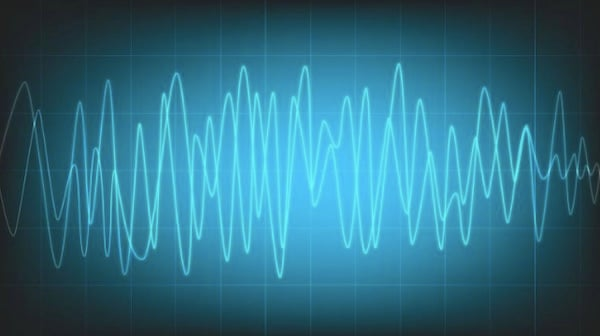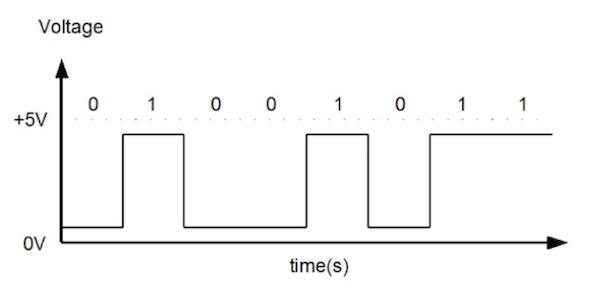# An Overview on Digital Signal Processing and Its ApplicationsNovember 01, 2019 by Sam Holland
ShareDigital signal processing, or DSP, is the process by which signals are manipulated, and it ultimately involves such manipulations taking different forms using mathematical tools.

Analogue signals (for the uninitiated, consider continuous time signals like sound, light intensity, temperature, and so on) can easily be converted to digital signals or discrete time signals through digital signal processing. This process is achieved by both the quantisation and sampling of the analogue signal in question.

A DSP system comprises the following:

• Data memory:  this stores the information to be processed by the DSP

• Compute engine: this carries out the mathematical computation of the information to be processed

• Program memory: this is where the program to be processed is stored

• Input/output: simply the DSP system’s connection ports to both take in and send out data and other information

### Computations in Digital Signal Processing

DSP has many mathematical computations required for the manipulation of signals. One major theorem, used in DSP to manipulate analogue signals during the analogue-to-digital conversion, is the sampling theorem.

The sampling theorem states that, to reconstruct a signal from its original form, the sampling frequency must be twice the highest frequency component in the signal.

In addition, DSP computation analysis can fall into 2 domains: time domain and frequency domain. Fourier transform is used to convert from time domain to frequency domain. It is easier for engineers to analyse the signal components in the frequency domain than in time domain. (Frequency domain analysis is also called spectrum analysis.)

Furthermore, to perform more analysis on signal, DSP incorporates the use of fast Fourier transforms—(named in honour of ground-breaking mathematician Joseph Fourier—(FFT), Discrete Fourier Transforms (DFT), and Discrete-Time Fourier Transform (DTFT). Any of these transforms can be used to study the spectral properties of signals. DFT can be used to analyse the spectrum representation of a finite length sampled signal.

Also, Z-transform, a mathematical tool used for analysis in DSP, can be used to solve problems in DSP filters. Z-transform has 2 basic properties: the linear property and the time-delay property.

Another notable point about computations in DSP is that it is possible to write algorithms for signal processing. (MATLAB, for instance, is a major tool used by engineers to write software for DSP hardware.)A line graph that represents digital signal changing with time. Image Credit: Plymouth University.

### Digital Signal Processing Filters

2 filters associated with DSP are the finite impulse response (FIR) filters and the infinite impulse response (IIR) filters. In FIR filters, the output values are the sum of finite numbers of weighted values of the input sequence. IIR filters, on the other hand, are recursive filters, the output is computed using previously computed values of the output signal as well as values of the input signal.

FIR filters are implemented using components such as adder, multiplier and unit delay operators: the adder operator is used to add 2 input signals; the multiplier multiplies signals; and the unit delay operator is used to pause operations by a unit of time. Z-transforms are used in the analysis of both FIR and IIR filters.

The 4 basic examples of FIR and IIR filters are low-pass filter, high-pass filter, bandstop filter, and bandpass filter. (Other examples of FIR filters include the FIR differentiator and Hilbert Transform.)

#### Linear Time-invariant Systems

DSP is used in the analysis of linear time-invariant (LTI) systems with the use of Z-transforms. A linear system obeys superposition and homogeneity rules. FIR filters and IIR filter-based systems are both linear and time-invariant.

Note that stability, moreover, is an important aspect when dealing with LTI systems: a system will be stable if the output is bounded for finite inputs. (Stable LTI systems are called bounded-input, bounded-output, aka BIBO, systems.)

#### Digital Signal Processing Software and Hardware

The development of DSP has led to the advent of complex algorithms deployed on hardware. An example of a DSP algorithm is the said FFT algorithms. Such algorithms can be deployed on field-programmable gate arrays, programmable logic devices, and application-specific integrated circuits.

DSP devices and microprocessors also incorporate the use of programming languages like C and C++ in their software architecture.

### Applications

Digital signal processing is used in many areas; after all, it is used in the common situation where, put simply, information in the real world is needed to be converted to digital formats and vice versa.

Accordingly, DSP is crucial for electrical and/or electronic engineers when working on DSP-based wireless communications, such as RF, alongside applications in communication engineering, avionics, speech processing, medicine, audio compression, image processing—and much more still.